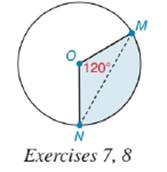Chapter 8.5, Problem 8EElementary Geometry For College St...

7th Edition
Alexander + 2 others
ISBN: 9781337614085

Solutions

Chapter
SectionElementary Geometry For College St...

7th Edition
Alexander + 2 others
ISBN: 9781337614085
Textbook Problem

If the area of the 1200 sector is 40 cm2 and the area of ∆ M O N is 16 cm2, what is the area of the segment bounded by M N - and M N ^ ?To determine

To find:

Thearea of the segment bounded by MN- and MN^

Explanation

Calculation:

Area of 1200 sector is given as 40 cm2.

Area of MON is given as 16 cm2.

From the diagram, it is clear that:

Area of 1200 sector = Area of MON+ Area of the segment bounded by MN- and MN^

Area of the segment bounded by MN- and MN^ = Area of 1200 sector - Area of MON

Let’s substitute the values of area of sector and MON in the above expression to find the area of

Still sussing out bartleby?

Check out a sample textbook solution.

See a sample solution

The Solution to Your Study Problems

Bartleby provides explanations to thousands of textbook problems written by our experts, many with advanced degrees!

Get Started

Define the terms population and sample, and explain the role of each in a research study.

Essentials of Statistics for The Behavioral Sciences (MindTap Course List)

For y = x3 ln x, y = a) x2(1 + 3 ln x) b) x3 + 3x2 (ln x) c) 3x d) 4x2(ln x)

Study Guide for Stewart's Single Variable Calculus: Early Transcendentals, 8th

Give the following tree diagram for an activity, what are the possible outcomes?

Finite Mathematics for the Managerial, Life, and Social Sciences